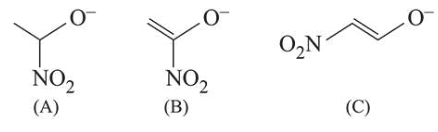# The correct order of stability for the following alkoxides is:

Question:

The correct order of stability for the following alkoxides is:1. $(\mathrm{B})>(\mathrm{A})>(\mathrm{C})$

2. $(\mathrm{C})>(\mathrm{B})>(\mathrm{A})$

3. $(\mathrm{C})>(\mathrm{A})>(\mathrm{B})$

4. $(\mathrm{B})>(\mathrm{C})>(\mathrm{A})$

Correct Option: , 2

Solution:

Electron withdrawing group like $\left(\mathrm{NO}_{2}\right)$ increase stability of alkoxide ion by dispersal of negative charge. In (B) and (C) structures negative charge is in conjugation with double bond and also stabilised by electron withdrawing effect of nitro group.